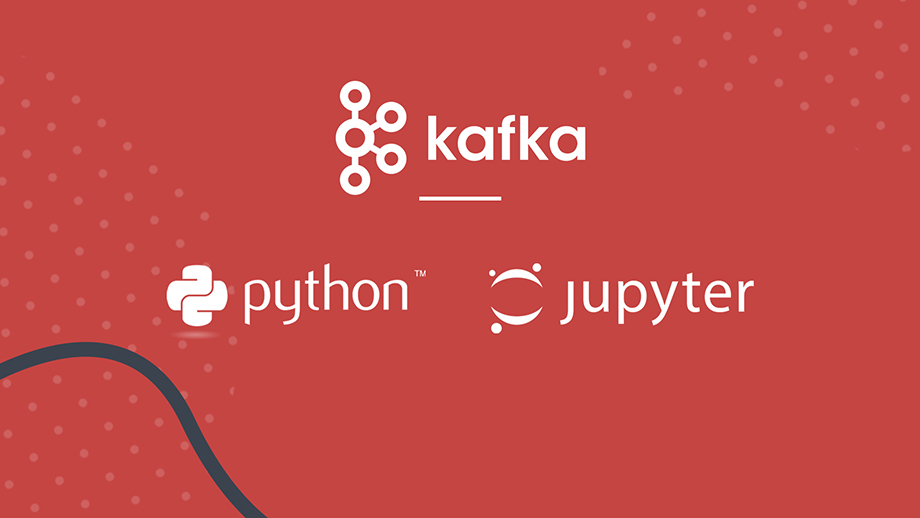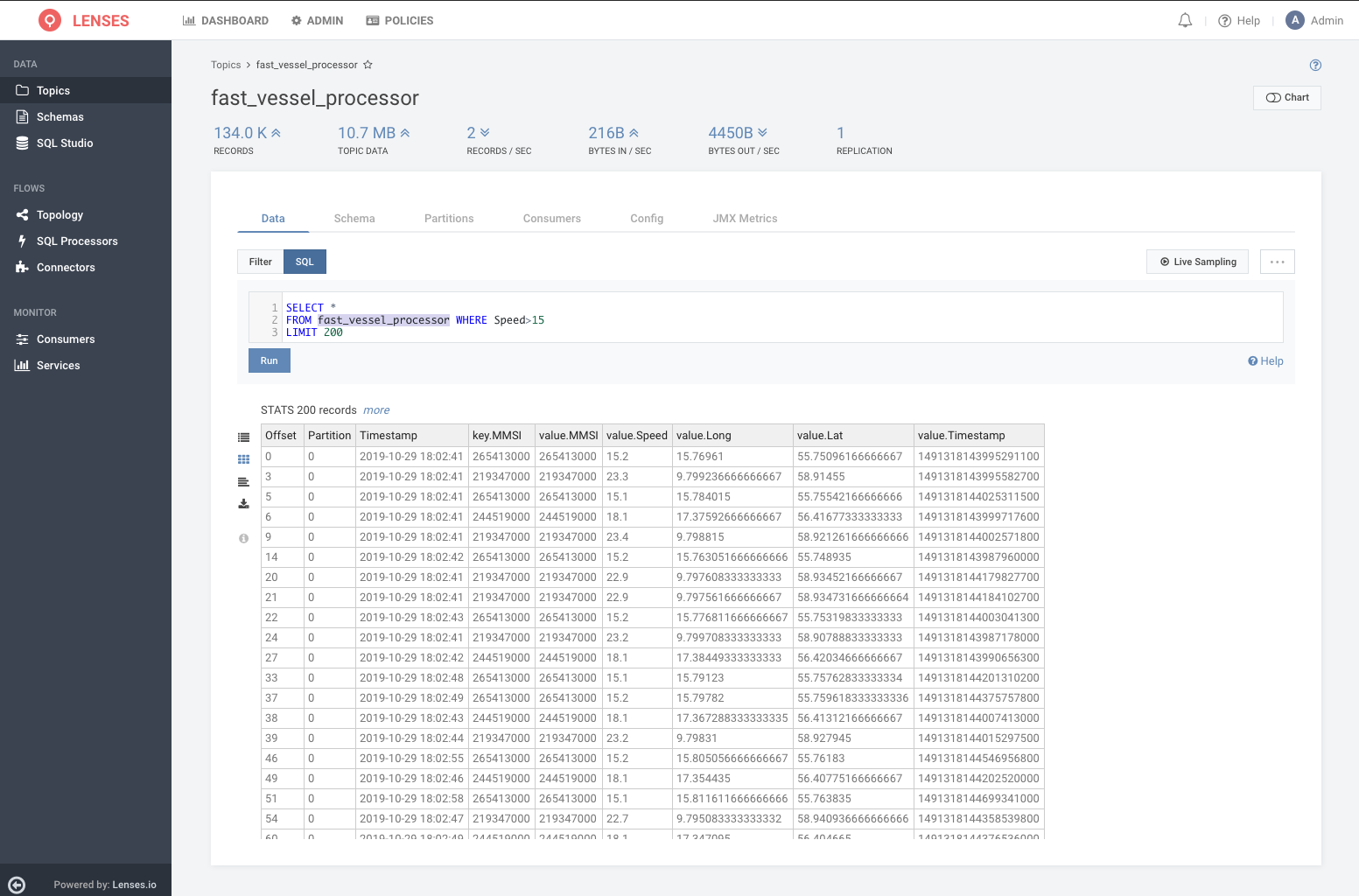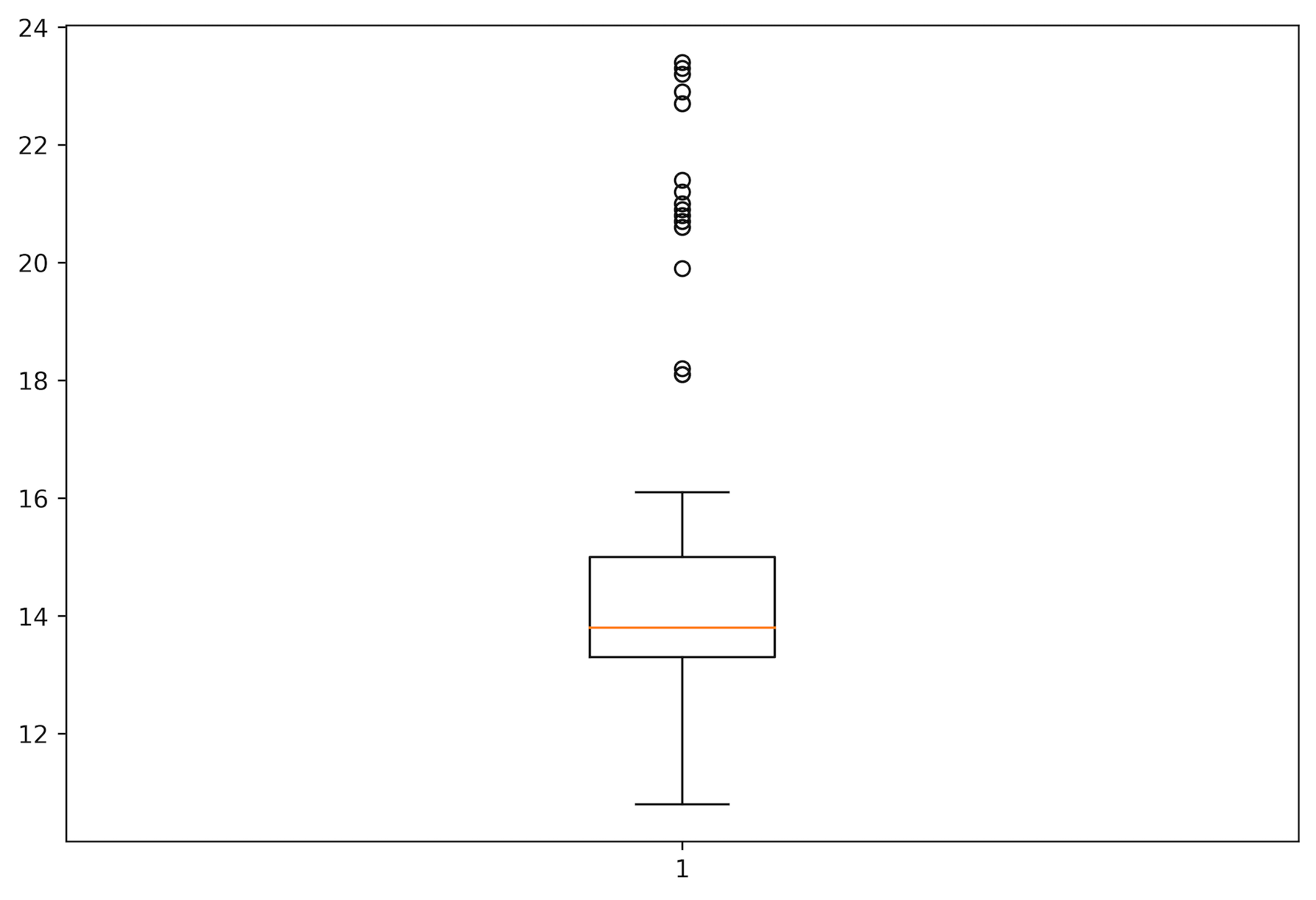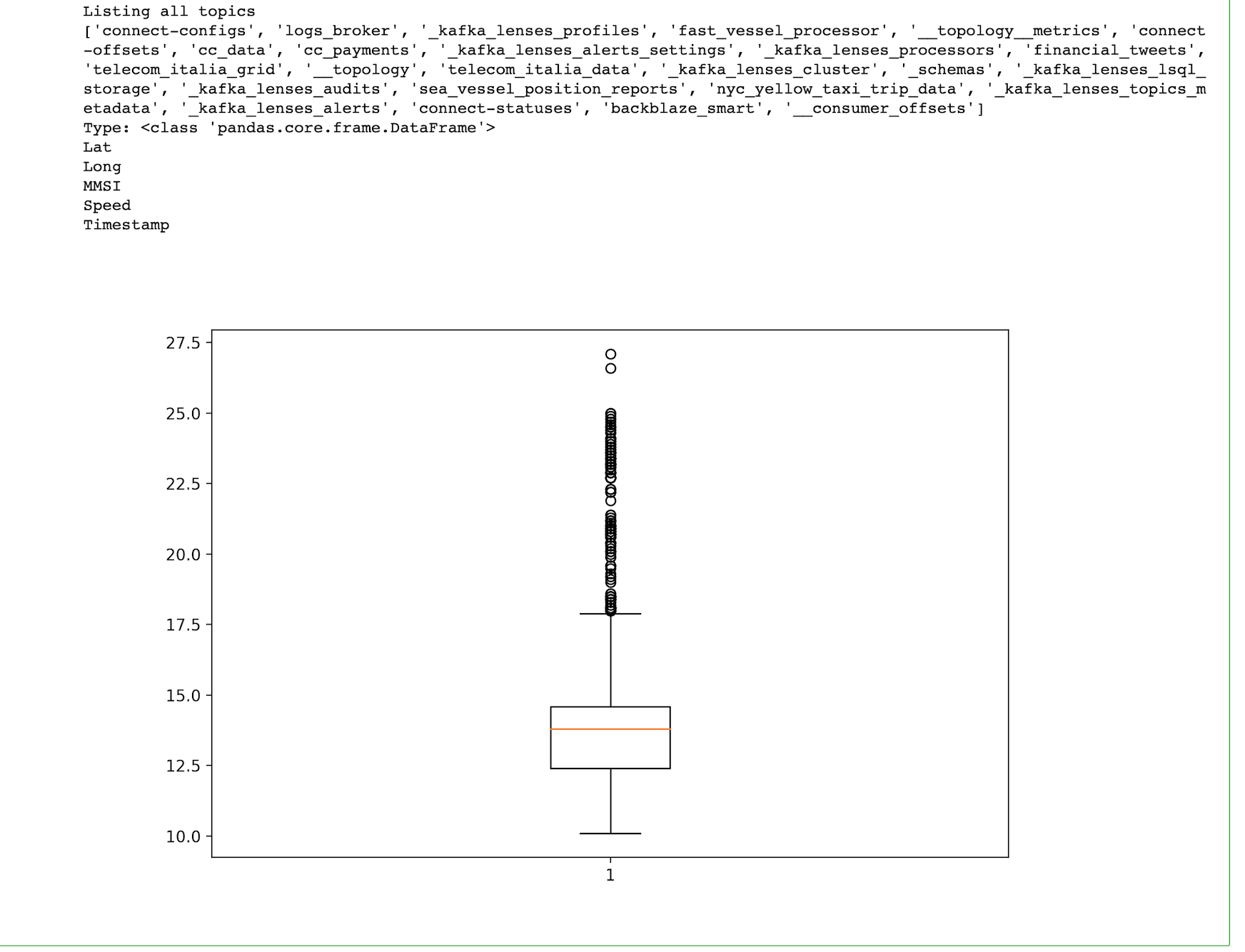# Using lenses-python to access streaming data in Apache Kafka

## How to use the lenses-python library to integrate streaming data in Apache Kafka into your python applications or Juypiter NotebooksOct 29, 2019As a secure portal for Apache Kafka, Lenses opens up access to streaming data to new usecases and users including data scientists, analysts and those not skilled on streaming technologies.

Data can be protected with role-based security, anonymised and queried with SQL and via a secure UI, CLI or API.

The Lenses `lenses-python` is a Python client that enables Python developers and data scientists to take advantage of the Rest and WebSocket endpoints Lenses exposes.

This blog outlines using the library to develop your own Lenses clients in Python 3. We will create two Python 3 utilities to create a box plot of the data found in a Kafka topic.

The first utility stores the output in a PNG file whereas the second utility uses a Jupyter Notebook to present the output.

## Pre-requisites

Download the free Lenses “Box”, a single container including an instance of Kafka, Lenses and sample streaming data which we’ll need for this walkthrough.

You are also going to need Lenses and a working Python 3 installation. If you want to use Jupyter, you will also need a working Jupyter installation.

## Installing Lenses Python Library

You can manually install `lenses-python` as follows:

`git clone https://github.com/landoop/lenses-python`
`cd lenses-python`
`pip3 install .`

Depending on your UNIX machine, you might need root privileges when executing the `pip3 install .` command.

After a successful installation, you can try the following to make sure that everything works as expected:

`python3`
`Python 3.7.4 (default, Jul  9 2019, 18:13:23)`
`[Clang 10.0.1 (clang-1001.0.46.4)] on darwin`
`Type "help", "copyright", "credits" or "license" for more information.`
`>>> from lenses_python.lenses import lenses`
`>>>`

## Connecting to Lenses using lenses-python

The presented Python 3 script will illustrate how you can connect to a running Lenses instance, which in this cases in a Lenses Box, using `lenses-python`.

The Python 3 code, which is saved in `conn_details.py`, is as follows:

`from lenses_python.lenses import lenses`
`data=lenses("http://127.0.0.1:3030","admin","admin")`
`print (data.GetCredentials())`

The parameters of the `lenses()` object, which is an alias for `lenses_python.lenses`, define the parameters of the connection, which are the URL of Lenses, the username and the password, respectively. What is returned is the parameters of the connection.

Executing `conn_details.py` will create the following kind of output:

`python3 conn_details.py`
`{'user': 'admin', 'schemaRegistryDelete': True, 'permissions': ['datapolicyread', 'nodata',`
`'tablestoragewrite', 'admin', 'alertswrite', 'tablestorageread', 'read', 'write',`
`'datapolicywrite', 'alertsread'], 'token': '00b5476b-fd34-4a70-b9df-f0f62d84f3cc'}`

If a Lenses instance is not available at the specified URL, you will get a `Connection refused` error message.

## Writing a Python 3 script

The presented Python 3 code will generate a box plot based on the data that is found in a Kafka topic called “fast_vessel_processor” (You can query the data in your instance via the UI with URL: localhost:3030/lenses/#/topics/fast_vessel_processor?f=sql)The Python 3 code, which is saved as `plot_data.py`, is as follows:

`from lenses_python.lenses import lenses`
`import pandas as pd`
`import numpy as np`
`import matplotlib as mpl`
`import matplotlib.pyplot as plt`
`# Create plot in PNG file`
`mpl.use('agg')`
`data=lenses("http://127.0.0.1:3030","admin","admin")`
`print("Listing all topics")`
`print(data.TopicsNames())`
`r = data.SqlHandler(`
`  'SELECT * FROM `fast_vessel_processor`',`
`  ['speed'])`
`# print(r)`
`print("Type:", type(r))`
`for i in r:`
`    print(i)`
`dataToPlot = []`
`for index, row in r.iterrows():`
`    dataToPlot.append(row['Speed'])`
`# Create a figure instance`
`fig = plt.figure(1, figsize=(9, 6), dpi=600)`
`# Create an axes instance`
`ax = fig.add_subplot(111)`
`# Create the boxplot`
`bp = ax.boxplot(dataToPlot)`
`# Save the figure`
`fig.savefig('boxplot.png', bbox_inches='tight')`

Executing `plot_data.py` will generate the following output:

`python3 plot_data.py`
`Listing all topics`
`['connect-configs', 'logs_broker', '_kafka_lenses_profiles', 'fast_vessel_processor',`
`'__topology__metrics', 'connect-offsets', 'cc_data', 'cc_payments', '_kafka_lenses_alerts_settings',`
`'_kafka_lenses_processors', 'financial_tweets', 'telecom_italia_grid', '__topology',`
`'_kafka_lenses_cluster', 'telecom_italia_data', '_schemas', '_kafka_lenses_lsql_storage',`
`'_kafka_lenses_audits', 'sea_vessel_position_reports', '_kafka_lenses_topics_metadata',`
`'nyc_yellow_taxi_trip_data', '_kafka_lenses_alerts', 'connect-statuses', 'backblaze_smart', '__consumer_offsets']`
`Type: <class 'pandas.core.frame.DataFrame'>`
`Lat`
`Long`
`MMSI`
`Speed`
`Timestamp`

So, `plot_data.py` lists all the available Kafka topics, the data type of the `r` variable and the names of the columns in the `fast_vessel_processor` Kafka topic.

Based on the data found in the Kafka topic used (`fast_vessel_processor`), the generated box plot will look as follows:## Using Jupyter

Jupyter Notebook allows you to create documents that contain live code, equations, visualizations and narrative text in a web browser.

The presented Python 3 code will create a box plot based on the data found in a Kafka topic inside a Jupyter notebook. The presented code is based on the Python 3 code of `plot_data.py`.

The Python 3 code used in the Jupyter notebook is as follows:

`from lenses_python.lenses import lenses`
`import pandas as pd`
`import numpy as np`
`from ipywidgets import interact`
`%matplotlib notebook`
`import matplotlib as mpl`
`import matplotlib.pyplot as plt`
`data=lenses("http://127.0.0.1:3030","admin","admin")`
`print("Listing all topics")`
`print(data.TopicsNames())`
`r = data.SqlHandler(`
`  'SELECT * FROM `fast_vessel_processor`',`
`  ['speed'])`
`print("Type:", type(r))`
`for i in r:`
`    print(i)`
`dataToPlot = []`
`for index, row in r.iterrows():`
`    dataToPlot.append(row['Speed'])`
`# Create a figure instance`
`fig = plt.figure(1, figsize=(9, 6))`
`# Create an axes instance`
`ax = fig.add_subplot(111)`
`# Create the boxplot`
`bp = ax.boxplot(dataToPlot)`

The output image of the previous code is the following:The output image is the same as the one generated by `plot_data.py` as both scripts use the same Kafka topic (`fast_vessel_processor`).

## Python Live Data Queries

The library also provides support for live streaming queries via SQL. See https://docs.lenses.io/dev/python-lib/index.html#continuous-queries for more details.

## Conclusions

The Lenses Python 3 library allows you to write handy and intelligent utilities that communicate with Lenses and take advantage of the power of the Python 3 programming language.

Want to start learning more about Kafka ?